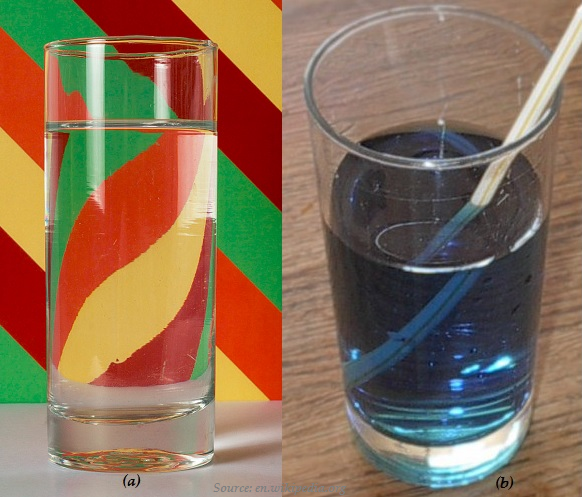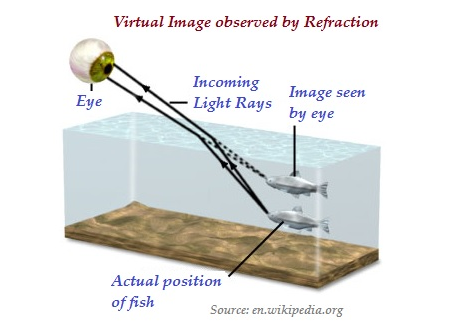# Physics - Refraction of Light

## Introduction

• Light, normally, travel along the straight-line paths in a transparent medium.

• When light is travelling obliquely from one medium to another, then the direction of propagation of light changes in the second medium, the phenomenon is known as refraction of light.

• In the image (a) given below, because of refraction in a glass of water, the image is flipped.• In the image (b) given above, the straw seems to be broken because of the refraction of light.• As shown in the image given above, because of the refraction in the water, the fish does not appear at its actual position rather a little above of its actual position.

## Laws of Refraction of Light

• Following are the significant laws of refraction of light−

• The incident ray, the refracted ray and the normal to the interface of two transparent media at the point of incidence, all lie in the same plane.

• The ratio of sine of angle of incidence to the sine of angle of refraction is a constant, for the light of a given color and for the given pair of media. This law is also known as Snell’s law of refraction.

• The constant value of the second medium with respect to the first is known as the refractive index.

## The Refractive Index

• In a given pair of media, the extent of the change in direction is expressed in terms of the refractive index.

• For a given pair of media, the value of the refractive index, depends upon the speed of light in the two media.

• The aptitude of a medium to refract light can be also expressed in terms of its optical density.

• The following table illustrates the absolute refractive index of some significant material media −

Material Medium Refractive Index
Air 1.0003
Ice 1.31
Water 1.33
Alcohol 1.36
Kerosene 1.44
Fused Quartz 1.46
Turpentine Oil 1.47
Rock Salt 1.54
Diamond 2.42